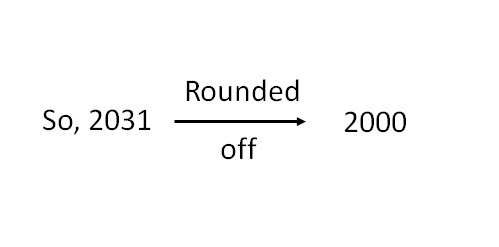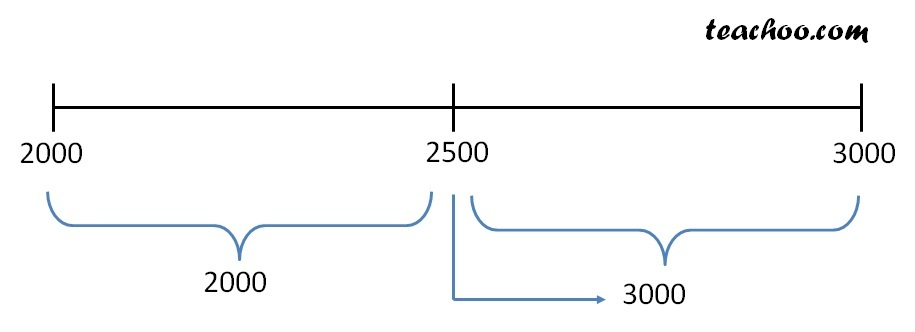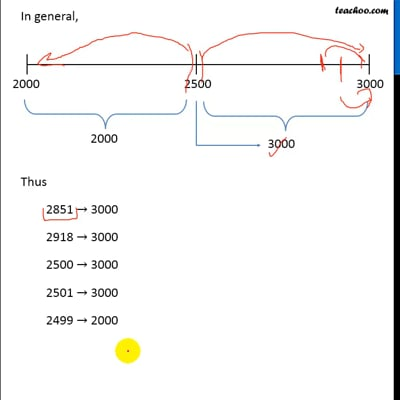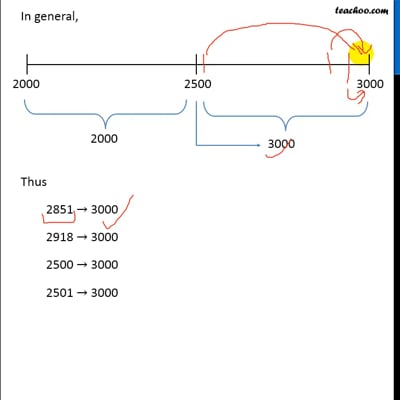Estimating and Rounding off

Chapter 1 Class 6 Knowing our Numbers
Concept wise

For number 2031,

Rounding off to nearest Thousand means

• We write number as 2000 or 3000

Since 2000 is near to 2031In general,Thus

2851 → 3000

2918 → 3000

2500 → 3000

2501 → 3000

2499 → 2000

2281 → 2000This video is only available for Teachoo black usersThis video is only available for Teachoo black users

### Transcript

Rounding off to nearest Thousand (1000) For number 2031, Rounding off to nearest Thousand means We write number as 2000 or 3000 Since 2000 is near to 2031 So, 2031 In general, Thus 2851 → 3000 2918 → 3000 2500 → 3000 2501 → 3000 2499 → 2000 2281 → 2000 General rule for rounding off General rule says For a number in tens, we round off to tens. For a number in hundred, we round off to hundred. For a number in thousands, we round off to thousands. Eg: 479 × 81 According to general rule, 479 is rounded off to nearest hundred ∴ 479 → 500 81 is rounded off to nearest ten ∴ 81 → 80 So, Estimated Product = 500 × 80 = 5 × 100 × 8 × 10 = (5 × 8) × 1000 = 40 × 1000 = 40000 Eg 2: 37 × 1889 According to general rule, 37 is rounded off to nearest ten ∴ 37 → 40 1889 is rounded off to nearest thousand ∴ 1889 → 2000 So, Estimated Product = 40 × 2000 = 4 × 10 × 2 × 1000 = (4 × 2) × 10000 = 8 × 10000 = 80000 Eg 3: 232 + 4821 According to general rule, 232 is rounded off to nearest hundred ∴ 232 → 200 4821 is rounded off to nearest thousand ∴ 4821 → 5000 So, Estimated Sum = 200 + 5000 = 5200 Eg 4: 1089 + 6871 According to general rule, 1089 is rounded off to nearest thousand, ∴ 1089 → 1000 6871 is rounded off to nearest thousand, ∴ 6871 → 7000 ∴ Estimated Sum = 1000 + 7000 = 8000 Eg 5: 9560 − 421 According to general rule, 9560 is rounded off to nearest thousand, ∴ 9560 → 10,000 421 is rounded off to nearest hundred, ∴ 421 → 400 ∴ Estimated Difference = 10,000 – 400 = 9600